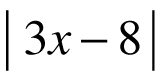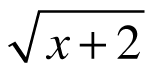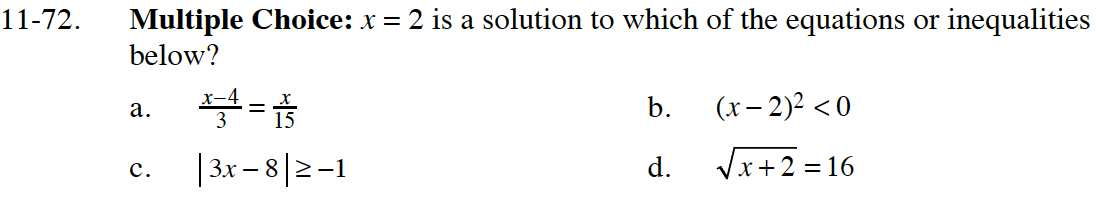Home > CC4 > Chapter 11 > Lesson 11.2.3 > Problem11-72

11-72.
1. Multiple Choice: x = 2 is a solution to which of the equations or inequalities below? Homework Help ✎

2.  A.B. (x − 2)2 < 0 C.≥ − 1 D.= 16One strategy is to substitute 2 for x in all of the equations and simplify.
If the equation or inequality is true, then x = 2 is a solution.